Lecture XIV

Physics 367

Nuclear Energy

Basics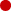The atom has a size of ___m.The nucleus has a size of 10-15m.

To put this in perspective:
If the atom is the size of a football field,
the nucleus is the size of a blade of grass!All nuclei are constructed with _____.

Nuclei are bound together by the strong force.

This force is about 100x greater than the electric force between two protons.

Both protons and neutrons are affected by the strong force.

The strong force is what binds protons and neutrons together in a nucleus.

The strong force is a short-range force.

Because protons and neutrons appear to act identically to the strong force [even though the proton is electrically charged!], they are often considered to be interchangeable and are called nucleons, or nuclear constituents. Only when the distinction is important do we call them protons or a neutrons.

We distinguish nuclei by:

A    mass number
Z    atomic number (number of protons)
N    number of neutrons

where A = Z + N

One interesting occurrence is that for existing nuclei as Z increases, N gets larger faster: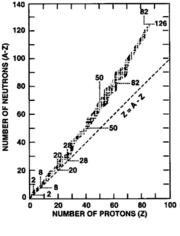The reason for this is: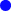protons repel each other [by what force?] and tend to make nuclei unstable.The neutrons act to screen the protons from each other making the nucleus stable...for effective screening there needs to be more neutrons than protons !

When protons and neutrons react together making bonds, they give up energy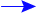nuclear binding energy.The binding energy increases as Z increases [the proton number]

The binding energy must increase with the number of nucleons because to get more together, they must collectively give up a large amount of energy.

Example: Hydrogen,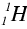, has no binding energy because it has only one nucleon in its nucleus. Uranium has a very large binding energy, partly because there are two hundred and thirty some nucleons inside the nucleus.

The actual binding energy is not as important as the average binding energy per nucleon. The average binding energy per nucleon is just the total binding energy divided by the number of nucleons. The average binding energy is used because each nucleon actually brought into a nucleus a different binding energy.

Think of balls falling into a hole.

The first few balls fall deeper into the hole than the last few.
The last ball in falls the least distance into the hole.

The situation for nucleons falling into the nuclear ``well'' is similar to this. Protons and neutrons are more stable inside the nucleus than outside.

Some nuclei have nucleons bound inside them more deeply than others. The average binding energy per nucleon then tells how deeply into the nuclear ``well'' the average nucleon has fallen.

How does the average binding energy per nucleon change as Z increases? We find that the average binding energy per nucleon first increases up to iron-56,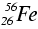, then decreases gradually as shown:The curve shows that nucleons can fall deeper into the nuclear ``well'' in two circumstances:If a nucleus to the right ofbreaks into smaller pieces, the nucleons inside the pieces are deeper inside the ``well'' than they had been in the original nucleus. - fissionIf two nuclei to the left ofcombine into a nucleus smaller than, the nucleons inside will find themselves deeper inside the ``well.'' - fusion

Nuclear fission

Nuclear fission is possible for nuclei with large A. It may happen spontaneously, but generally it occurs because some activation energy was sent into the nucleus. To get a nuclear reaction to start, even one that produces useful energy, it is often necessary to give some energy to the system. The energy provided to start a reaction going is called the nuclear activation energy.

Since there are more neutrons per proton in the large-A nucleus than in the breakup products, nuclear fission will involve the release of the extra neutrons. There are many ways for any nucleus to break up, or fission. After the activation energy has been brought inside the nucleus by a slow neutron that interacts with the nucleus, the nucleus acts in a way similar to taffy being pulled.

The amount of taffy held in each hand is determined randomly. Sometimes the right hand will be holding a lot more taffy than the left; and sometimes the reverse is true.

In a similar way, when a neutron comes in bringing activation energy, the nucleus is pulled about. It will break up in random ways.Consider as a specific example the fission of uranium-235. One possible outcome is that the fission will produce strontium-97 and xenon-137:Note: the A's and Z's add up on both sides of the arrow if the reaction is to proceed!

The binding energy curve can be used to find the average binding energy per nucleon for the nuclei above.

Uranium-236 has a binding energy of 7.6 MeV/nucleon
Strontium-97 has a binding energy of 8.6 MeV/nucleon
Xenon-137 has a binding energy of 8.4 MeV/nucleon.

Thus, the total binding energy:

Uranium-236 is 236 x (7.6 MeV) = 1794 MeV
Strontium-97 is 97 x (8.6 MeV) = 834 MeV
Xenon-137 is 137 x (8.4 MeV) = 1151 MeV
Neutrons have a total binding energy of 0

Thus, the nucleons give up the difference between the final total binding energy and the initial binding energy:

final:        1975 MeV = 834 MeV + 1151 MeV + 0
initial:      1794 MeV
difference: 191 MeV

The energy appears as kinetic energy of the nuclei and the neutrons, as- and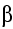- particles, and as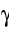-radiation, as well as neutrinos, massless particles that can carry away energy.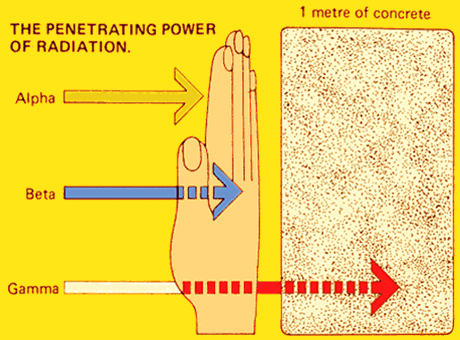Energy Release

The forces holding the nuclei together are very strong, thus there is a lot of energy released. There is 100 million (108) times as much energy available from the fission of a uranium-235 nucleus as from the burning of a carbon atom.

Recall a coal-fired plant uses 100 kg of coal per second. In a day, this amounts to 8,640,000 kg, or 8,640 tonnes, of coal. That would take about 350 to 400 railroad cars of coal a day. A fission power reactor producing the same amount of energy uses about 3 kg of uranium-235 per day. To produce this amount of energy from nuclear fission, almost 1020 fissions per second must take place.

In addition to the large amount of energy given off in each fission, neutrons are produced in the fission. On the average, 2.43 neutrons are produced from each fission.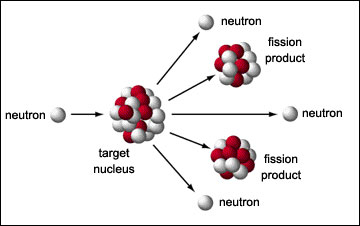The extra neutrons produced may go on to cause another nucleus to fission, and another, and another; so the number of fissioning nuclei can increase rapidly with time.

That is, there can be a nuclear chain reaction, just as there can be a chemical chain reaction. The rate of a chemical reaction can be controlled; similarly, the rate of the nuclear reaction can be controlled.

Nuclear fission can be used to produce electricity at slow rates, or can be used as a bomb, in which case there is an explosively fast chain reaction. The proportion of uranium-235 [or other fissile material] is the chief determinant of whether the chain reaction will be explosive or not. Also important is the ability to let neutrons escape or to capture them.

 Uranium-235 Concentrations Natural Uranium 0.7% Nuclear Reactor Fuel 4.0% Atomic Bomb 90%

Problem of the day

It is a project proposed by LANL to produce reactor fuel and electricity by repeated underground thermonuclear explosion. The proposal is to detonate 2 devices per day in a mile-deep cavity.

How will this work?

The nuclear explosive will heat water (1 million tons) in the sealed cavity to produce superheated steam that would power a regular generating plant on the surface and also breed fuel in the cavity.

If each device has 25 kiloton of explosive at 10% efficiency then we get a total energy output of:

(25 kton/device)(2 devices/day)=50 kton/day

from Table 7.1 for dynamite

(5.4x106J/kg)(0.91x106kg/kton) = 4.91x1012J/kton

(50 kton/day)(4.91x1012J/kton)(0.10) = 2.5x1013J/day

(2.5x1013J/day)/(3.6x106J/kWh) = 6.8x106kWh/day

which yields

(6.8x106kWh/day)(day/24hr) = 280 MW